Function Repository Resource:

# Fractran

Interpret the FRACTRAN esoteric programming language

Contributed by: Daniel Sanchez and Jan Mangaldan
 ResourceFunction["Fractran"][prog,n] runs the FRACTRAN program prog on the integer n.

## Details and Options

FRACTRAN is an esoteric programming language invented by the mathematician John H. Conway.
The result of a FRACTRAN execution is a list of integers.
A FRACTRAN program can have one of the following forms:
 FRACTRAN-1 program {f1,f2,…} where each fi is a positive fraction FRACTRAN-n program {…,{fl1→l1,fl2→l2,…},…} where flm are positive fractions and each lm is an integer between 1 and n (the length of the list)
The FRACTRAN-1 program ResourceFunction["Fractran"][{f1,f2,,fi,},n] repeatedly multiplies the integer at any stage (initially n) against the earliest fraction fi in the list that yields an integer product, stopping the execution when there is no such fi.
The FRACTRAN-n program ResourceFunction["Fractran"][{,{fl1l1,fl2l2,},},n] repeatedly multiplies the integer at any stage (initially n) against the earliest fraction flm in line l (initially 1) that yields an integer product, then proceeds to the line indicated by lm, stopping the execution when there is no such flm.
The first element of the resulting list is always the starting integer n.
If ResourceFunction["Fractran"] terminates early, the last element of the resulting list is 0.
ResourceFunction["Fractran"] has the following options:
 MaxIterations Infinity maximum number of iterations "MaxResults" Infinity maximum number of integers in the result "SelectionCriterion" Automatic test to determine whether an integer should be included in the result "ShowProgramLines" False if set to True, show the program line currently being executed
The FRACTRAN language can be considered a type of register machine where: 1) The integer n stores the contents of the registers in the exponents of prime factors of n. For example 34992 = 24×37 represents a register state in which the register "2" holds the value 4 and the register "3" the value 7. 2) Each positive fraction fi in a program represents an instruction that operates on the integer n. The instruction is executed only if fi × n yields an integer product, otherwise, a negative value will be stored in the register state n. For example, the fraction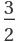can operate on 24×37 resulting in 23×38, but not on 311 as the final register state would be 2-1×312.

## Examples

### Basic Examples (3)

Run a simple FRACTRAN program representing integer addition, where given an input of the form 2a3b the program will have a + b encoded as 3a+b in the last positive integer of the resulting list:

 In:=Out=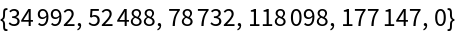In:=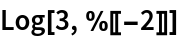Out=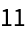The following program represents integer subtraction, where given the input of the form 2a3b the program will have a - b encoded as 2a-b (for a>b) in the last positive integer of the resulting list:

 In:=Out=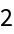Use John Conway's PRIMEGAME program to compute prime numbers encoded as powers of 2 if the program is started at 2:

 In:=Out=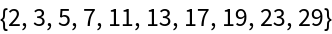In:=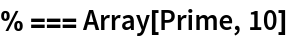Out=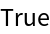### Scope (2)

#### FRACTRAN-1 program (1)

Define addition by using the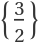FRACTRAN-1 program:

 In:=Out=#### FRACTRAN-n program (1)

The following FRACTRAN-n program corresponds to multiplication, where given the input of the form 3a7b the program will stop when 2a b is computed:

 In:=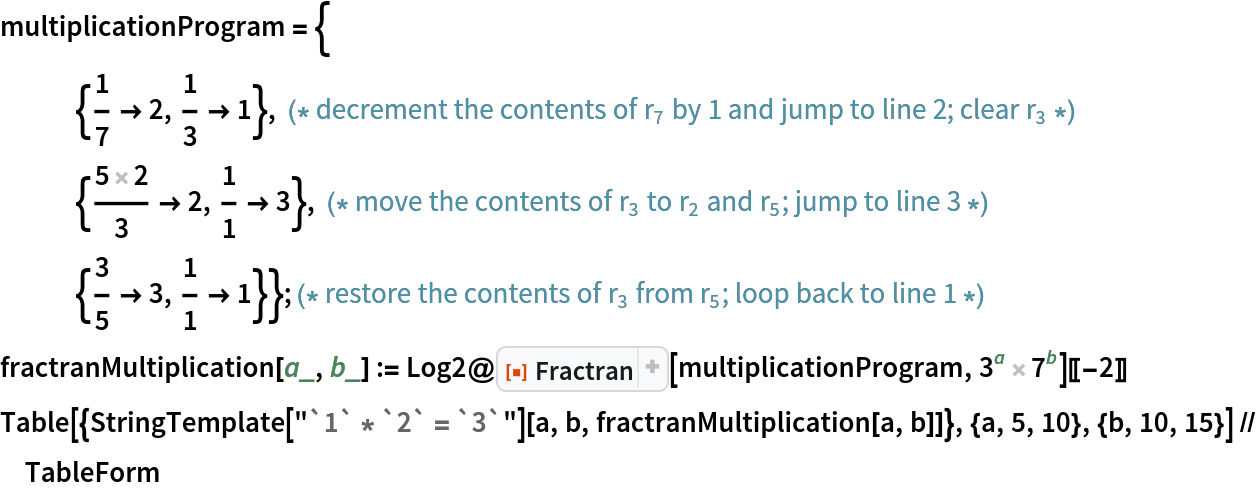Out=### Options (6)

#### MaxIterations (1)

MaxIterations specifies the maximum number of steps to take in running the Fractran program:

 In:=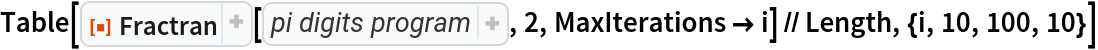Out=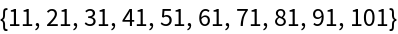#### MaxResults (2)

"MaxResults" specifies the maximum number of integers to be returned, excluding the initial condition:

 In:=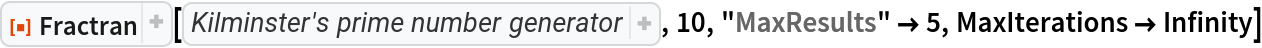Out=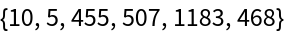This option is most commonly used with "SelectionCriterion":

 In:=Out=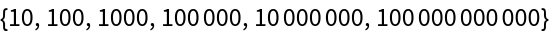#### SelectionCriterion (1)

For "SelectionCriterion" crit, all integers ni of the resulting list for which crit[ni] is True are picked:

 In:=Out=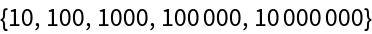#### ShowProgramLines (2)

If "ShowProgramLines" set to True, append the program line currently being executed:

 In:=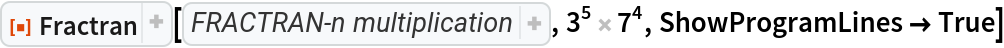Out=This option is mainly used alongside FRACTRAN-n programs. For FRACTRAN-1 programs, the line shown is always 1:

 In:=Out=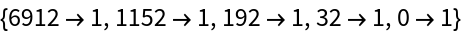### Possible Issues (1)

The default value of the MaxIterations is Infinity and as such, special care should be taken when the Fractran program does not halt:

 In:=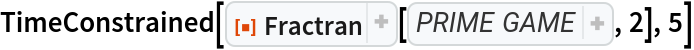Out=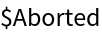### Neat Examples (2)

Kilminster's prime number generator:

 In:=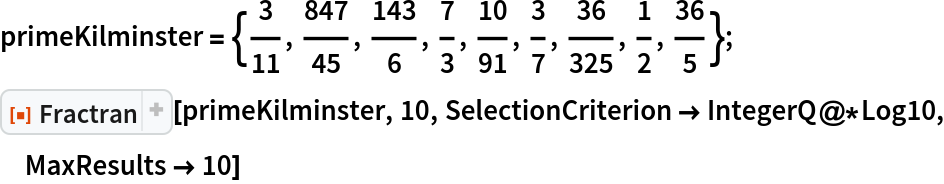Out=Use IntegerLength to get the result:

 In:=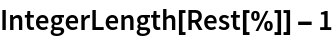Out=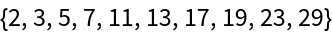Calculate the Hamming weight H[a] of the binary expansion of a, where if given the input 2a its output is 13H[a]:

 In:=Out=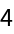Compare it with a custom Hamming weight defined in terms of HammingDistance:

 In:=Out=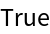Daniel Sanchez

## Requirements

Wolfram Language 12.2 (December 2020) or above

## Version History

• 1.0.0 – 18 January 2021